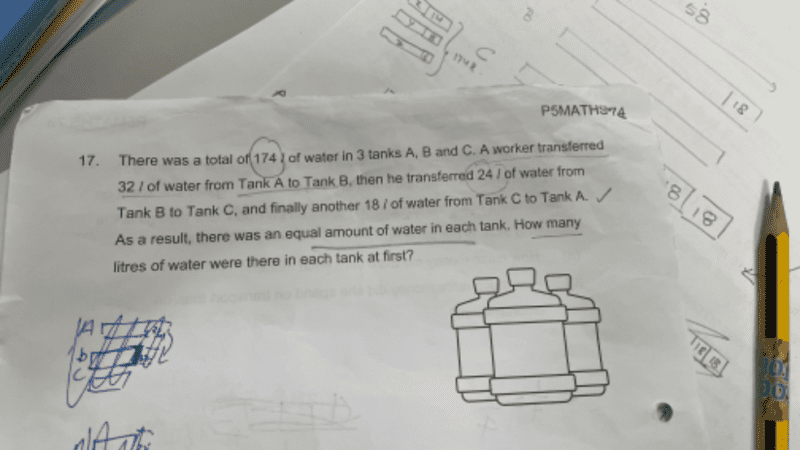# QuestionA + B + C = 174 … (1)

Now, B + 32 – 24 = C + 24 – 18 = A – 32 + 18
B + 8 = C + 6 = A – 14

A = B + 8 + 14 = B + 22 … (2)

B = C + 6 – 8 = C – 2 … (3)

Substituting (3) into (2):
A = C – 2 + 22 = C + 20 … (4)

Substituting (3) and (4) into (1):
(C + 20) + (C – 2) + C = 174
3C + 18 = 174
3C = 156
C = 52 #

So B = C – 2 = 52 -2 = 50 #

And A = B + 22 = 50 + 22 = 72 #

0 Replies 1 Like